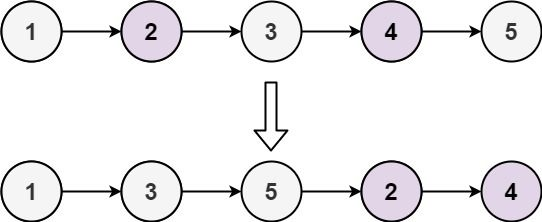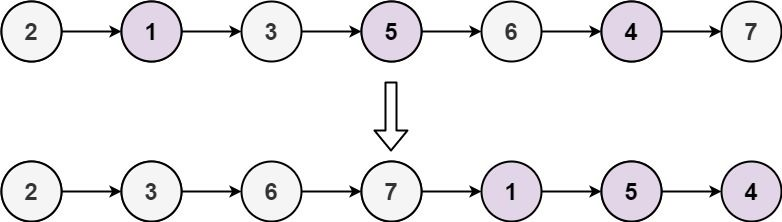# Question

Formatted question description: https://leetcode.ca/all/328.html

Given the head of a singly linked list, group all the nodes with odd indices together followed by the nodes with even indices, and return the reordered list.

The first node is considered odd, and the second node is even, and so on.

Note that the relative order inside both the even and odd groups should remain as it was in the input.

You must solve the problem in O(1) extra space complexity and O(n) time complexity.

Example 1:Input: head = [1,2,3,4,5]
Output: [1,3,5,2,4]


Example 2:Input: head = [2,1,3,5,6,4,7]
Output: [2,3,6,7,1,5,4]


Constraints:

• The number of nodes in the linked list is in the range [0, 104].
• -106 <= Node.val <= 106

# Algorithm

Two parity pointers are used to point to the starting position of the even node respectively, and a separate pointer even_head is needed to save the starting position of the even node.

Then point the odd node to the next one of the even node (it must be the odd node), and move this odd node one step later.

Then point the even node to the next odd node (it must be an even node), this even node is moved one step later, and so on until the end.

At this time, the linked list of the separated even node is connected after the linked list of the odd node.

# Code

• 
/**
* public class ListNode {
*     int val;
*     ListNode next;
*     ListNode(int x) { val = x; }
* }
*/

class Solution {

}

boolean isEven = true; // start from 2nd

while (current != null) {

if (isEven) {
evenPrev.next = current;
evenPrev = current;
} else {
oddPrev.next = current;
oddPrev = current;
}

// flip
isEven = !isEven;
current = current.next;
}

// @note: cut last even->odd, or else loop
evenPrev.next = null;

// connect

}
}
}

############

/**
* public class ListNode {
*     int val;
*     ListNode next;
*     ListNode() {}
*     ListNode(int val) { this.val = val; }
*     ListNode(int val, ListNode next) { this.val = val; this.next = next; }
* }
*/
class Solution {
}
while (even != null && even.next != null) {
odd.next = even.next;
odd = odd.next;
even.next = odd.next;
even = even.next;
}
}
}

• // OJ: https://leetcode.com/problems/odd-even-linked-list/
// Time: O(N)
// Space: O(1)
class Solution {
public:
if (odd) {
} else {
}
}
evenTail->next = NULL;
}
};

• # Definition for singly-linked list.
# class ListNode:
#     def __init__(self, val=0, next=None):
#         self.val = val
#         self.next = next
class Solution:
def oddEvenList(self, head: ListNode) -> ListNode:
while even and even.next:
odd.next = even.next
odd = odd.next
even.next = odd.next
even = even.next

############

# class ListNode(object):
#     def __init__(self, x):
#         self.val = x
#         self.next = None

class Solution(object):
"""
:rtype: ListNode
"""
o = odd = ListNode(-1)
e = even = ListNode(-1)
isOdd = True
while p:
if isOdd:
o.next = p
o = o.next
isOdd = False
else:
e.next = p
isOdd = True
e = e.next
p = p.next
e.next = None
o.next = even.next
return odd.next


• /**
* type ListNode struct {
*     Val int
*     Next *ListNode
* }
*/
}
for even != nil && even.Next != nil {
odd.Next = even.Next
odd = odd.Next
even.Next = odd.Next
even = even.Next
}
}

• /**
* class ListNode {
*     val: number
*     next: ListNode | null
*     constructor(val?: number, next?: ListNode | null) {
*         this.val = (val===undefined ? 0 : val)
*         this.next = (next===undefined ? null : next)
*     }
* }
*/

function oddEvenList(head: ListNode | null): ListNode | null {
while (even != null && even.next != null) {
odd.next = even.next;
odd = odd.next;
even.next = odd.next;
even = even.next;
}